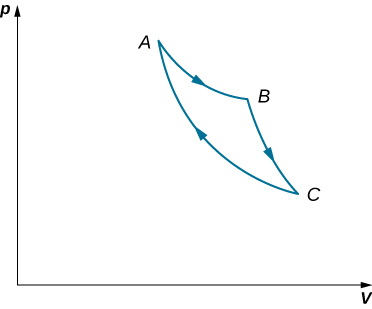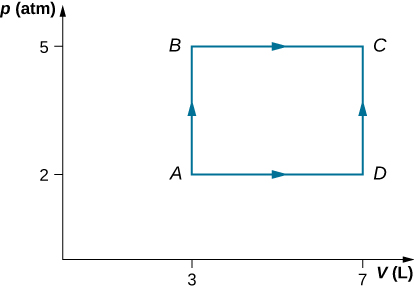3.4 Thermodynamic processes  (Page 4/11)

 Page 4 / 11

Many other processes also occur that do not fit into any of these four categories.

View this site to set up your own process in a pV diagram. See if you can calculate the values predicted by the simulation for heat, work, and change in internal energy.

Summary

• The thermal behavior of a system is described in terms of thermodynamic variables. For an ideal gas, these variables are pressure, volume, temperature, and number of molecules or moles of the gas.
• For systems in thermodynamic equilibrium, the thermodynamic variables are related by an equation of state.
• A heat reservoir is so large that when it exchanges heat with other systems, its temperature does not change.
• A quasi-static process takes place so slowly that the system involved is always in thermodynamic equilibrium.
• A reversible process is one that can be made to retrace its path and both the temperature and pressure are uniform throughout the system.
• There are several types of thermodynamic processes, including (a) isothermal, where the system’s temperature is constant; (b) adiabatic, where no heat is exchanged by the system; (c) isobaric, where the system’s pressure is constant; and (d) isochoric, where the system’s volume is constant.
• As a consequence of the first law of thermodymanics, here is a summary of the thermodymaic processes: (a) isothermal: $\text{Δ}{E}_{\text{int}}=0,Q=W;$ (b) adiabatic: $Q=0,\text{Δ}{E}_{\text{int}}=\text{−}W;$ (c) isobaric: $\text{Δ}{E}_{\text{int}}=Q-W;$ and (d) isochoric: $W=0,\text{Δ}{E}_{\text{int}}=Q.$

Conceptual questions

When a gas expands isothermally, it does work. What is the source of energy needed to do this work?

The system must be in contact with a heat source that allows heat to flow into the system.

If the pressure and volume of a system are given, is the temperature always uniquely determined?

It is unlikely that a process can be isothermal unless it is a very slow process. Explain why. Is the same true for isobaric and isochoric processes? Explain your answer.

Isothermal processes must be slow to make sure that as heat is transferred, the temperature does not change. Even for isobaric and isochoric processes, the system must be in thermal equilibrium with slow changes of thermodynamic variables.

Problems

Two moles of a monatomic ideal gas at (5 MPa, 5 L) is expanded isothermally until the volume is doubled (step 1). Then it is cooled isochorically until the pressure is 1 MPa (step 2). The temperature drops in this process. The gas is now compressed isothermally until its volume is back to 5 L, but its pressure is now 2 MPa (step 3). Finally, the gas is heated isochorically to return to the initial state (step 4). (a) Draw the four processes in the pV plane. (b) Find the total work done by the gas.

Consider a transformation from point A to B in a two-step process. First, the pressure is lowered from 3 MPa at point A to a pressure of 1 MPa, while keeping the volume at 2 L by cooling the system. The state reached is labeled C . Then the system is heated at a constant pressure to reach a volume of 6 L in the state B . (a) Find the amount of work done on the ACB path. (b) Find the amount of heat exchanged by the system when it goes from A to B on the ACB path. (c) Compare the change in the internal energy when the AB process occurs adiabatically with the AB change through the two-step process on the ACB path.

a. 1660 J; b. −2730 J; c. It does not depend on the process.

Consider a cylinder with a movable piston containing n moles of an ideal gas. The entire apparatus is immersed in a constant temperature bath of temperature T kelvin. The piston is then pushed slowly so that the pressure of the gas changes quasi-statically from ${p}_{1}$ to ${p}_{2}$ at constant temperature T. Find the work done by the gas in terms of n, R, T, ${p}_{1},$ and ${p}_{2}.$

An ideal gas expands isothermally along AB and does 700 J of work (see below). (a) How much heat does the gas exchange along AB? (b) The gas then expands adiabatically along BC and does 400 J of work. When the gas returns to A along CA, it exhausts 100 J of heat to its surroundings. How much work is done on the gas along this path?a. 700 J; b. 500 J

Consider the processes shown below. In the processes AB and BC, 3600 J and 2400 J of heat are added to the system, respectively. (a) Find the work done in each of the processes AB, BC, AD, and DC. (b) Find the internal energy change in processes AB and BC. (c) Find the internal energy difference between states C and A. (d) Find the total heat added in the ADC process. (e) From the information give, can you find the heat added in process AD? Why or why not?Two moles of helium gas are placed in a cylindrical container with a piston. The gas is at room temperature $25\phantom{\rule{0.2em}{0ex}}\text{°}\text{C}$ and under a pressure of $3.0\phantom{\rule{0.2em}{0ex}}×\phantom{\rule{0.2em}{0ex}}{10}^{5}\phantom{\rule{0.2em}{0ex}}\text{Pa}\text{.}$ When the pressure from the outside is decreased while keeping the temperature the same as the room temperature, the volume of the gas doubles. (a) Find the work the external agent does on the gas in the process. (b) Find the heat exchanged by the gas and indicate whether the gas takes in or gives up heat. Assume ideal gas behavior.

a. −3 400 J; b. 3400 J enters the gas

An amount of n moles of a monatomic ideal gas in a conducting container with a movable piston is placed in a large thermal heat bath at temperature ${T}_{1}$ and the gas is allowed to come to equilibrium. After the equilibrium is reached, the pressure on the piston is lowered so that the gas expands at constant temperature. The process is continued quasi-statically until the final pressure is 4/3 of the initial pressure ${p}_{1}.$ (a) Find the change in the internal energy of the gas. (b) Find the work done by the gas. (c) Find the heat exchanged by the gas, and indicate, whether the gas takes in or gives up heat.

a cold body of 100°C and a hot body is of 100°F . Transfer heat = ?
you are given two metal spheres mounted on portable insulating support. Find a way to give them equal and opposite charges. you may use a glass rod rubbed with silk but may not touch it to the spheres. Do the spheres have to be of equal size for your method to work?
in the 2nd example, for chapter 8.2 on page 3/3, I don't understand where the value 48uC comes from, I just couldn't get that value in my calculator.
are you talking about the capacitance combination problem
sam
please write the problem or send a snap of th page....I don't have the book in my vicinity.
SABYASACHI
what is electromagnetic force. do electric and magnetic force happen differently
yes
yes
Pranay
why
Godson
how?
Godson
derived the electric potential due to disk of charge
how can we derived potential electric due to the disk
aron
how can you derived electric potential of a disk
aron
how can you derived electric potential due to disk
aron
where is response?
aron
what is difference between heat and temperature?
temperature is the measure of degree of hotness or coldness. on the other hand, heat is the form of energy, which causes temperature. So we can safely say, heat is the reason and temperature is its consequence.
SABYASACHI
how many liquid metals do we have
do we have gasses as metals
Jeffery
who knows should please tell us
yes...gallium & cesium
Idris
Hg is liquid. No metal gasses at standard temp and pressure
Shane
I don't ever understand any of this formulae
which formula
How to determine a temperature scale
what is the formula for absolute error
Nyro
define kelvin planck statement
using p-v diagram, explain what takes place during each four processes of a carnot cycle
Masia
using p-v diagram, explain what takes place during each four processes of a carnot cycle
Masia
state zeroths law of thermodynamics
The zeroth law of thermodynamics states that if twothermodynamic systems are each in thermal equilibrium with a third one, then they are in thermal equilibrium with each other
Seza
zeroth law of thermodynamic state that when a body A and B are in thermal equilibrium to body C ,a state is attained when body A,B and C are in thermal equilibrium.
akanbi
sorry guys I got no any idea on the law can someone help me please?
SNAHR
hi
Muhsin
The zeroth law of thermodynamics states that if two thermodynamic systems are each in thermal equilibrium with a third one, then they are in thermal equilibrium with each other. Accordingly, thermal equilibrium between systems is a transitive relation. Two systems are said to be in the relation of
Muhsin
I can help u Snahr as possible
Vinayaka
it simply states that, if two bodies are separately in thermal equilibrium with a third body, then those two bodies will also be in thermal equilibrium with each other
Vinayaka
What mass of steam of 100 degree celcius must be mixed with 150g of ice at 0 degree celcius, in a thermally insulated container, to produce liquid water at 50 degree celcius
sorry I dont know
Bamidele
thank you
Emmanuel
What is the pressure?
SHREESH
To convert 0°C ice to 0°c water. Q=M*s=150g*334J/g=50100 J.......... Now 0° water to 50° water... Q=M*s*dt=150g*4.186J/g*50= 31395 J....... Which adds upto 81495 J..... This is amount of heat the steam has to carry. 81495= M *s=M*2230J/g..therefore.....M=36.54g of steam
SHREESH
This is at 1 atm
SHREESH
If there is change in pressure u can refer to the steam table ....
SHREESH
instrument for measuring highest temperature of a body is?
Thermometer
Umar
how does beryllium decay occur
Photon?
Umar
state the first law of thermodynamics
Its state that "energy can neither be created nor destroyed but can be transformed from one form to another. "
Ayodamola
what about the other laws can anyone here help with it please
Sandy
The second law of thermodynamics states that the entropy of any isolated system always increases. The third law of thermodynamics states that the entropy of a system approaches a constant value as the temperature approaches absolute zero.
sahil
The first law is very simple to understand by its equation. The law states that "total energy in thermodynamic sytem is always constant" i.e d¶=du+dw where d¶=total heat du=internal energy dw=workdone... PLEASE REFER TO THE BOOKS FOR MORE UNDERSTANDING OF THE CONCEPT.
EliaByBy